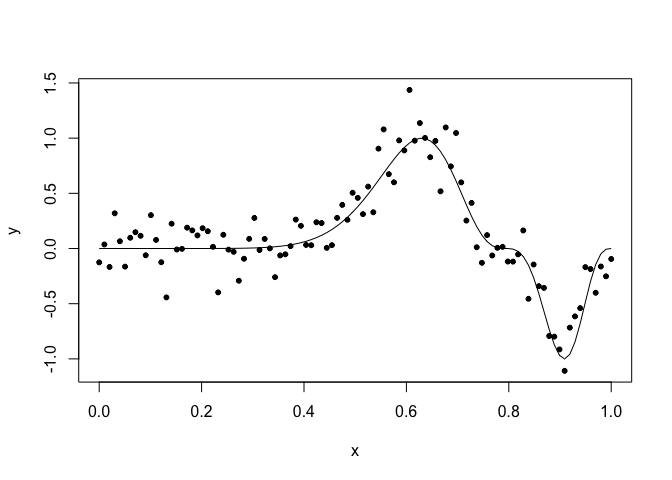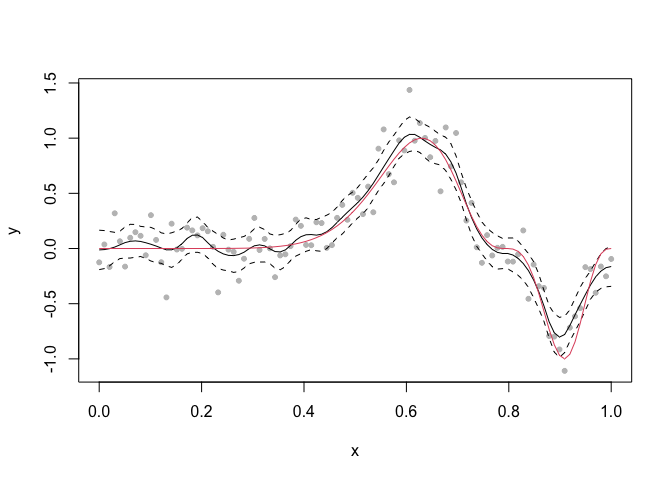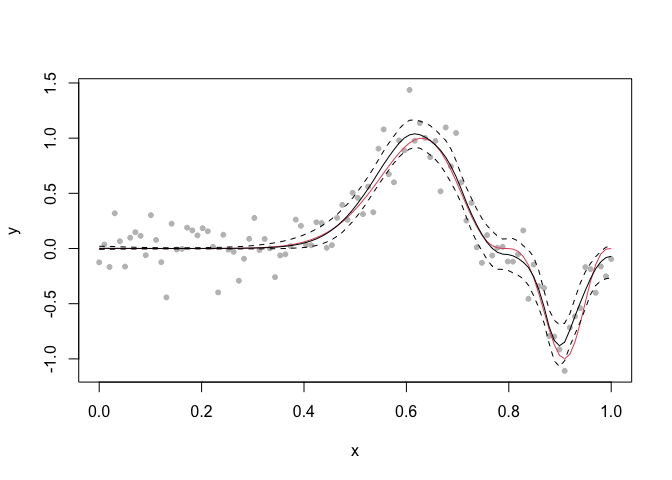# Non-stationary smoothing with Gaussian process regression using INLA

Julian Faraway 21 September 2020

See the introduction for more about INLA. See an example of Gaussian process regression. The construction is detailed in our book.

Load the packages (you may need to install the brinla package)

``````library(INLA)
library(brinla)
``````

# Data

We simulate some data with a known true function.

``````set.seed(1)
n <- 100
x <- seq(0, 1, length = n)
f.true <- (sin(2 * pi * (x)^3))^3
y <- f.true + rnorm(n, sd = 0.2)
td <- data.frame(y = y, x = x, f.true)
``````

and plot it:

``````plot(y ~ x, td)
lines(f.true ~ x, td)
``````This function is challenging to fit because of the varying amount of smoothness. You can see examples of various smoothing methods applied to this data in (Faraway 2016).

# GP fitting

The default fit uses priors based on the SD of the response and the range of the predictor to motivate sensible priors.

``````gpmod = bri.gpr(td\$x, td\$y)
``````

We can plot the resulting fit and 95% credible bands

``````plot(y ~ x, td, col = gray(0.75))
lines(gpmod\$xout, gpmod\$mean)
lines(gpmod\$xout, gpmod\$lcb, lty=2)
lines(gpmod\$xout, gpmod\$ucb, lty=2)
lines(f.true ~ x, td, col=2)
``````On the right end of the function, the fit is too rough. On the left end, it is too smooth. The minimum at 0.9 is underestimated and the true function is even outside the credibility bands in this region. The problem is that the standard method uses the same amount of smoothness everywhere.

# Variable smoothness

We can allow the smoothness to vary. A description of this can be found in (Lindgren and Rue 2015) and also in our book. We have implemented this in our `brinla` package:

``````fg <- bri.nonstat(td\$x, td\$y)
plot(y ~ x, td, col = gray(0.75))
lines(f.true ~ x, td, col = 2)
lines(fg\$xout, fg\$lcb, lty=2)
lines(fg\$xout, fg\$ucb, lty=2)
lines(fg\$xout, fg\$mean)
``````We achieve a smoother fit on the left but keep the less smooth fit on the right.

# Package versions

``````sessionInfo()
``````
``````R version 4.0.2 (2020-06-22)
Platform: x86_64-apple-darwin17.0 (64-bit)
Running under: macOS Catalina 10.15.6

Matrix products: default
BLAS:   /Library/Frameworks/R.framework/Versions/4.0/Resources/lib/libRblas.dylib
LAPACK: /Library/Frameworks/R.framework/Versions/4.0/Resources/lib/libRlapack.dylib

locale:
 en_GB.UTF-8/en_GB.UTF-8/en_GB.UTF-8/C/en_GB.UTF-8/en_GB.UTF-8

attached base packages:
 parallel  stats     graphics  grDevices utils     datasets  methods   base

other attached packages:
 brinla_0.1.0  INLA_20.03.17 foreach_1.5.0 sp_1.4-2      Matrix_1.2-18 knitr_1.29

loaded via a namespace (and not attached):
 codetools_0.2-16     lattice_0.20-41      digest_0.6.25        grid_4.0.2           MatrixModels_0.4-1
 magrittr_1.5         evaluate_0.14        rlang_0.4.7          stringi_1.4.6        rmarkdown_2.3
 splines_4.0.2        iterators_1.0.12     tools_4.0.2          stringr_1.4.0        xfun_0.16
 yaml_2.2.1           compiler_4.0.2       htmltools_0.5.0.9000
``````
Faraway, Julian. 2016. *Extending the Linear Model with R: Generalized Linear, Mixed Effects and Nonparametric Regression Models*. 2nd ed. London: Chapman & Hall.
Lindgren, Finn, and Havard Rue. 2015. “Bayesian Spatial Modelling with R-INLA.” *Journal of Statistical Software* 63 (19): 1–25.